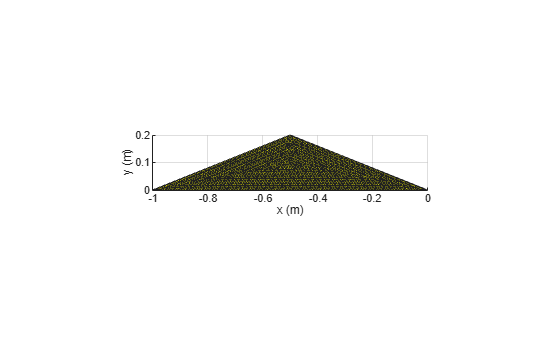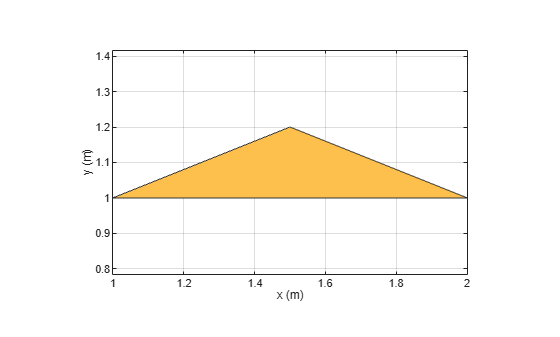# antenna.Polygon

Create polygon on X-Y plane

## Description

Use the `antenna.Polygon` object to create a polygonal board shape centered at the origin and on the X-Y plane. You can use `antenna.Polygon` to create single-layer or multilayered antennas using `pcbStack`.

## Creation

### Syntax

``polygon = antenna.Polygon``
``polygon = antenna.Polygon(Name,Value)``

### Description

````polygon = antenna.Polygon` creates a polygonal board shape centered at the origin and on the X-Y plane.```

example

````polygon = antenna.Polygon(Name,Value)` sets properties using one or more name-value pair. For example, ```polygon = antenna.Polygon('Name','mypolygonboard')``` creates a polygon board shape of the name `'mypolygonboard'`. Enclose each property name in quotes.```

## Properties

expand all

Name of the polygon board shape, specified a character vector or string.

Example: `'Name','Polygon1'`

Example: `polygon.Name = 'Polygon1'`

Data Types: `char` | `string`

Cartesian coordinates of polygon vertices, specified as a N-by-3 matrix with each element measured in meters, N being the number of points.

Example: ```'Vertices',[-1 0 0;-0.5 0.2 0;0 0 0]```

Example: ```polygon.Vertices = [-1 0 0;-0.5 0.2 0;0 0 0]```

Data Types: `double`

## Object Functions

 `add` Boolean unite operation on two shapes `area` Calculate area of shape in square meters `subtract` Boolean subtraction operation on two shapes `intersect` Boolean intersection operation on two shapes `rotate` Rotate shape about axis and angle `rotateX` Rotate shape about x-axis and angle `rotateY` Rotate shape about y-axis and angle `rotateZ` Rotate shape about z-axis and angle `translate` Move shape to new location `show` Display antenna or array structure; display shape as filled patch `mesh` Mesh properties of metal or dielectric antenna or array structure `removeHoles` Remove holes from shape `removeSlivers` Remove sliver outliers from boundary of shape

## Examples

collapse all

Create a polygon using `antenna.Polygon` with vertices at `[-1 0 0;-0.5 0.2 0;0 0 0]` and view it.

`p = antenna.Polygon('Vertices', [-1 0 0;-0.5 0.2 0;0 0 0])`
```p = Polygon with properties: Name: 'mypolygon' Vertices: [3x3 double] ```
```show(p) axis equal```Mesh the polygon and view it.

`mesh(p,0.2)`Move the polygon to a new location on the X-Y plane.

```translate(p,[2,1,0]) axis equal```